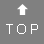# Letters and numbers - How to calculate the cross sum

For example turn letters from your full name to find your destiny number:

 1 2 3 4 5 6 7 8 9 a b c d e f g h i j k l m n o p q r s t u v w x y z
Example:
• A person with the name Holly Grace Snyder would find their Destiny Number by calculating first Holly (8 + 6 + 3 + 3 + 7 = 27), reducing that to a single-digit number (2 + 7 = 9)
• Then you calculate Grace (7 + 9 + 1 + 3 + 5 = 25), reducing that to a single-digit number (2 + 5 = 7)
• Then you calculate Snyder (1 + 5 + 7 + 4 + 5 + 9 = 31), reducing that to a single-digit number (3 + 1 = 4)
• Then you add the three single-digit numbers together (9 + 7 + 4 = 20), and reduce that to a single-digit number (2 + 0 = 2)
Holly Grace Snyder would therefore have a destiny number of 2

You can also easily calculate the cross sum here.

Henrik - 2016-03-28 23:31:23
mod - 2016-04-16 09:16:10
Hi Henrik,just reduce your result to a single-digit number: 3+8 = 11, 1+1=2 ...

Total 2 posts